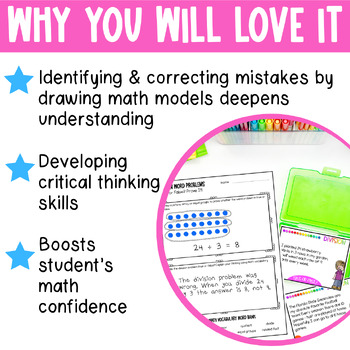# Division Word Problems Task Cards Error Analysis Math3rd - 4th, Homeschool
Subjects
Standards
Resource Type
Formats Included
• PDF
Pages
12 pages

#### Also included in

1. Do your students need more in depth mathematical tasks? These error analysis equation word problems will be a great resource in your math classroom. Students will analyze multiplication and division word problems to find the errors, then use math models to make their case. Explaining their thinking
\$6.75
\$9.00
Save \$2.25
2. This 3rd Grade mega bundle of error analysis math task cards will give you rigorous standards based year long math practice for your students! This resource includes multiplication, division, fractions, geometry, and much more! Print once, laminate and they are ready to go! Each skill has error ana
\$45.60
\$57.00
Save \$11.40

### Description

Make division practice fun and challenging for your 3rd grade students! These error analysis division word problems will be a important part of math lessons and practice in math centers. Your third grade students will analyze division word problems to find the errors and use math models to make their case in writing.

CLICK HERE to SAVE 20% on Division and Multiplication Word Problems Bundle!

PLEASE SEE PREVIEW FOR A CLOSER LOOK!

Included in this resource:

♦ 36 Division Word Problem Task Cards

♦ 1 Prove It Worksheet

♦ 1 Challenge a Classmate Worksheet

This resource is also available in a bundle pack:
Multiplication & Division Word Problems Task Cards - Error Analysis Bundle Pack

This resource is perfect for:

• Whole Group Instruction

• Small Group Instruction

• Centers

• Formative Assessment

• Partner Work

• Bellwork

You Might Also Like These Other Division Resources:

"Don't forget to leave feedback -you will receive TPT credits that you can use toward future purchases!

Thank you for visiting Teaching in the Heart of Florida!

Total Pages
12 pages
Included
Teaching Duration
Other
Report this Resource to TpT
Reported resources will be reviewed by our team. Report this resource to let us know if this resource violates TpT’s content guidelines.

### Standards

to see state-specific standards (only available in the US).
Interpret products of whole numbers, e.g., interpret 5 × 7 as the total number of objects in 5 groups of 7 objects each. For example, describe a context in which a total number of objects can be expressed as 5 × 7.
Interpret whole-number quotients of whole numbers, e.g., interpret 56 ÷ 8 as the number of objects in each share when 56 objects are partitioned equally into 8 shares, or as a number of shares when 56 objects are partitioned into equal shares of 8 objects each. For example, describe a context in which a number of shares or a number of groups can be expressed as 56 ÷ 8.
Use multiplication and division within 100 to solve word problems in situations involving equal groups, arrays, and measurement quantities, e.g., by using drawings and equations with a symbol for the unknown number to represent the problem.
Solve two-step word problems using the four operations. Represent these problems using equations with a letter standing for the unknown quantity. Assess the reasonableness of answers using mental computation and estimation strategies including rounding.
Interpret a multiplication equation as a comparison, e.g., interpret 35 = 5 × 7 as a statement that 35 is 5 times as many as 7 and 7 times as many as 5. Represent verbal statements of multiplicative comparisons as multiplication equations.